# The four particles of the figure are connected with tubes of negligible mass. A. Determine the...

The four particles of the figure are connected with tubes of negligible mass.
A. Determine the moment of inertia of this system relative to the axes x,y and z
B. Calculate the rotational kinetic energy of this system in each of the chaos of the previous part.y(m) 3.00 kg 2.00 kg 6.00 m x(m) 4.00 m 2.00 kg 4.00 kg

We need at least 10 more requests to produce the answer.

0 / 10 have requested this problem solution

The more requests, the faster the answer.

All students who have requested the answer will be notified once they are available.

#### Earn Coin

Coins can be redeemed for fabulous gifts.

Similar Homework Help Questions
• ### The four particles shown below are connected by rigid rods of negligible mass where y1-6.60 m....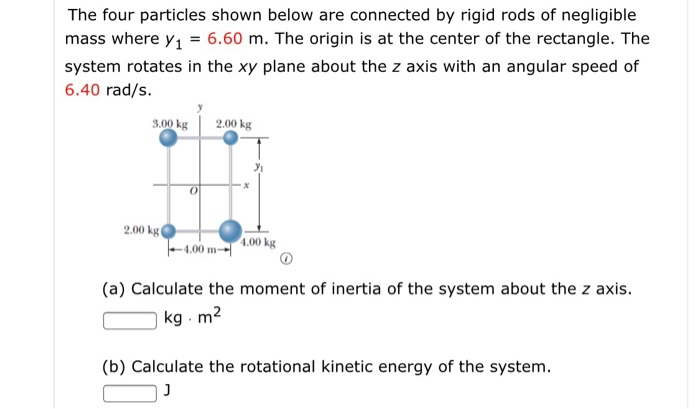The four particles shown below are connected by rigid rods of negligible mass where y1-6.60 m. The origin is at the center of the rectangle. The system rotates in the xy plane about the z axis with an angular speed of 6.40 rad/s. 3.00 kg 2.00 kg 0 2.00 kg 1.00 kg 4.00 m (a) Calculate the moment of inertia of the system about the z axis kg m2 (b) Calculate the rotational kinetic energy of the system.

• ### The figure shows three particles connected on a rod, of negligible mass, along the y axis....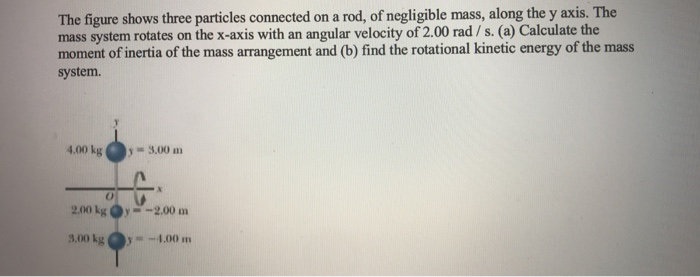The figure shows three particles connected on a rod, of negligible mass, along the y axis. The mass system rotates on the x-axis with an angular velocity of 2.00 rad / s. (a) Calculate the moment of inertia of the mass arrangement and (b) find the rotational kinetic energy of the mass system 4.00 kg , -9.00 m 2004 -2,00 m 3,00 kg y -4.00 m

• ### 9. [-/2 Points] DETAILS SERPSE10 10.7.OP.021. MY NOTES PRACTICE ANOTHER The four particles shown below are...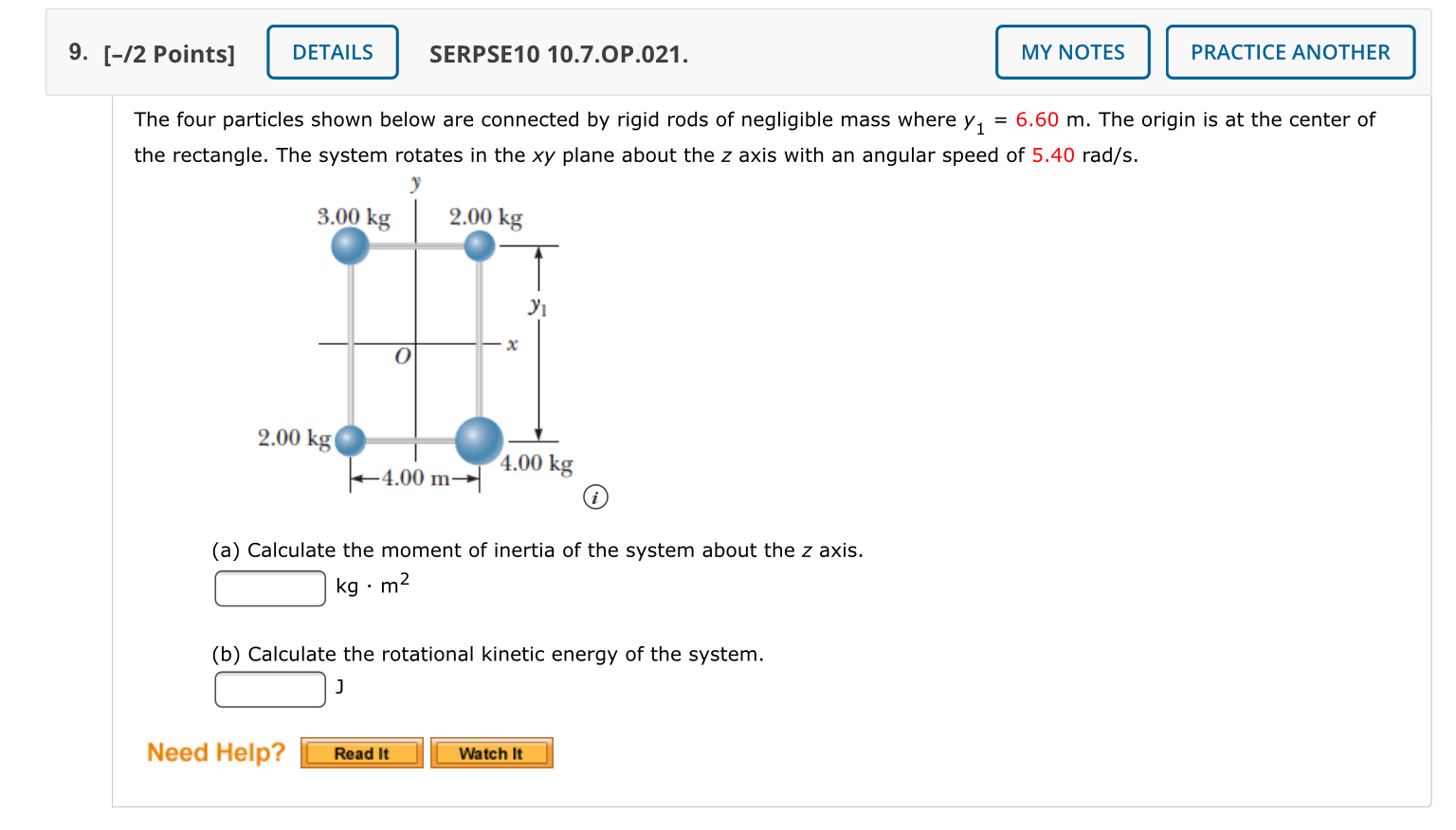9. [-/2 Points] DETAILS SERPSE10 10.7.OP.021. MY NOTES PRACTICE ANOTHER The four particles shown below are connected by rigid rods of negligible mass where y1 = 6.60 m. The origin is at the center of the rectangle. The system rotates in the xy plane about the z axis with an angular speed of 5.40 rad/s. 3.00 kg 2.00 kg Yi X 0 2.00 kg 4.00 kg - 4.00 m- (a) Calculate the moment of inertia of the system about the...

• ### rods of negligible mass lying along the y axis connect three particles. The system rotates about...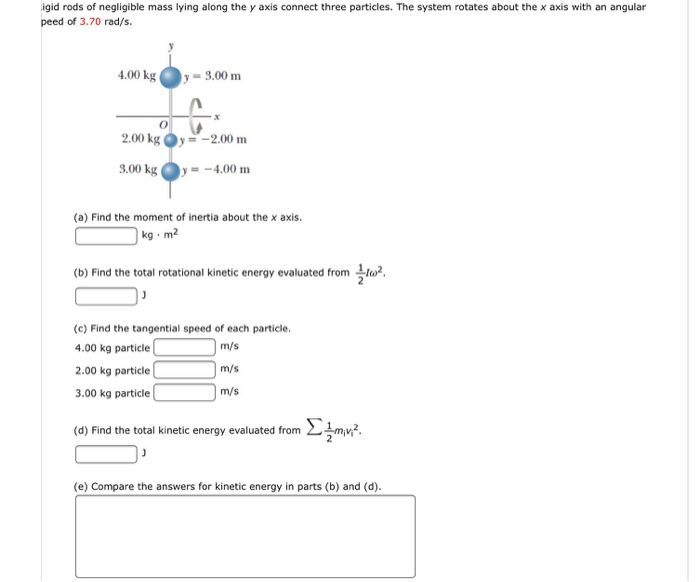rods of negligible mass lying along the y axis connect three particles. The system rotates about the x axis with an angular peed of 3.70 rad/s. (a) Find the moment of inertia about the x axis. kg middot m^2 (b) Find the total rotational kinetic energy evaluated from 1/2 I omega^2. J (c) Find the tangential speed of each particle. 4.00 kg particle m/s 2.00 kg particle m/s 3.00 kg particle m/s (d) Find the total kinetic energy evaluated from...

• ### step by step. box in answer please Rigid rods of negligible mass lying along the y...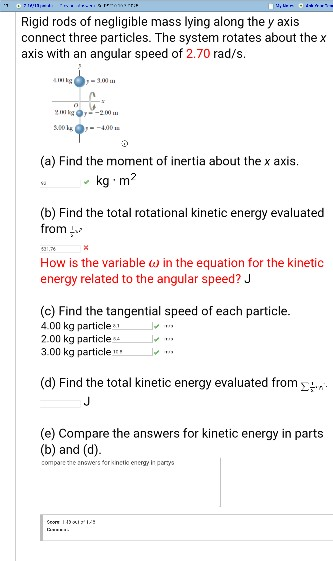step by step. box in answer please Rigid rods of negligible mass lying along the y axis connect three particles. The system rotates about the x axis with an angular speed of 2.70 rad/s (a) Find the moment of inertia about the x axis kg m (b) Find the total rotational kinetic energy evaluated from How is the variable ω in the equation for the kinetic energy related to the angular speed? J (c) Find the tangential speed of each...

• ### The four particles shown in Fig. connected by rigid rods. The or igin is at the...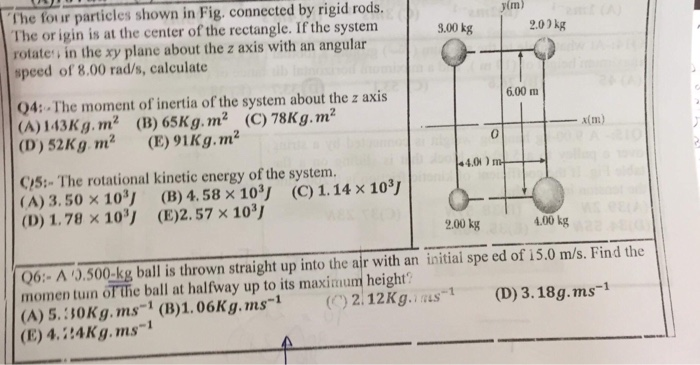The four particles shown in Fig. connected by rigid rods. The or igin is at the center of the rectangle. If the system rotate in the xy plane about the z axis with an angular speed of 8.00 rad/s, calculate yim) 9.00 kg 2.00kg 04: The moment of inertia of the system about the z axis (A) 143Kg. m2 (B) 65Kg.m2 (C) 78Kg. m2 (D) 52Kg m2 (E) 91Kg.m2 6.00 m x(m) 0 CS: The rotational kinetic energy of the...

• ### The four particles shown in Fig. conriected by rigid rods. The origin is at the center...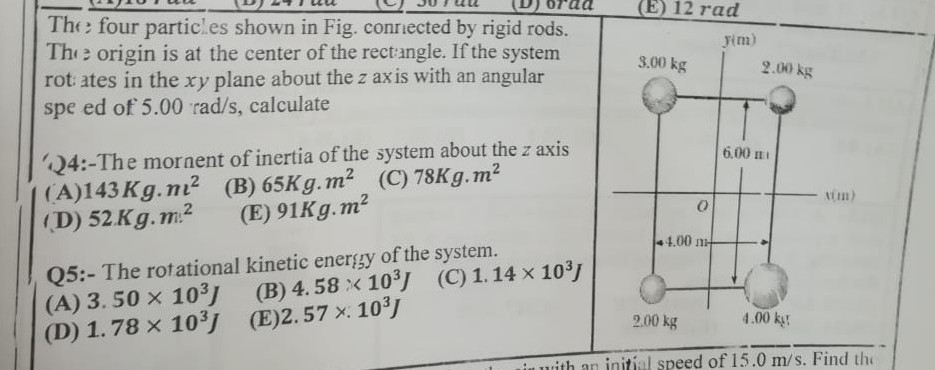The four particles shown in Fig. conriected by rigid rods. The origin is at the center of the rectangle. If the system rot ates in the xy plane about the z axis with an angular spe ed of 5.00 rad/s, calculate Fim) 3.00 kg 2.00 kg 4:-The mornent of inertia of the system about the z axis (A)143 Kg ni2 (B) 65Kg.m2 (C) 78Kg.m2 (D) 52 Kg.m2 (E) 91Kg.m2 6.00 ni im) 4.00mH Q5:- The rotational kinetic energy of the...

• ### Four particles, one at each of the four corners of a square with 2.5-m-long edges, are connected by mass less rods

Four particles, one at each of the four corners of a square with 2.5-m-long edges, are connected by mass less rods. The masses of the particles are m1 = m3 = 2.0 kg and m2 = m4 = 7.0 kg. Find the moment of inertia of the system about the z axis.Rigid rods of negligible mass lying along the y axisconnect three particles (Fig. P10.20). The system rotates about thex axis with an angular speedof 3.00 rad/s.Figure P10.20(a) Find the moment of inertia about thex axis.kg·m2Find the total rotational kinetic energy evaluated from 1/2I2. J(b) Find the tangential speed of each particle. m/s (4.00 kgparticle) m/s (2.00 kgparticle) m/s (3.00 kgparticle)Find the total kinetic energy evaluated from mivi2/2. J

• ### Rigid rods of negligible mass lying along the y axis connect three particles. The system rotates...Rigid rods of negligible mass lying along the y axis connect three particles. The system rotates about the x axis with anangular speed of 1.50 rad/s.(b) Find the tangential speed of each particle.m/s (4.00 kg particle)m/s (2.00 kg particle)m/s (3.00 kg particle)